# DSPace Based Implementation of DRFOC Using Hysteresis Stator Flux Controllers for IM

DSPace Based Implementation of DRFOC Using Hysteresis Stator Flux Controllers for IM

Abdesslam Lokriti Issam Salhi* Said Doubabi

Laboratory of Informatics, Systems, Electrical, Networks and Telecommunications, Sultan Moulay Slimane University Beni mellal, Morocco

Laboratory of Electric Systems and Telecommunications, Cadi Ayyad University, BP 549, Av Abdelkarim Elkhattabi, Gueliz, Marrakesh, Morocco

Corresponding Author Email:
isalhi@yahoo.fr
Page:
61-66
|
DOI:
https://doi.org/10.18280/ejee.210110
29 December 2018
|
Accepted:
3 February 2019
|
Published:
15 April 2019
| Citation

OPEN ACCESS

Abstract:

The purpose of this paper is to prove that Induction Motor (IM) torque drive based on Direct Rotor Field Oriented Control (DRFOC) could be achieved using no currents controllers and no PWM block. In fact, we propose, after an inverse Park rotation applied on direct and quadratic stator flux components, to control both stator flux components, real and imaginary, through two hysteresis controllers. Therefore, a switching table is established. It’s independent from sector determination, does not introduce zero voltage vectors and with reduced size compared to Takahashi’s switching table. Thus, a new approach to realize DRFOC is provided. The proposed DRFOC is validated by practical implementation on a DSPace 1104 board, for a 1.5 kW IM.

Keywords:

induction motor, direct rotor field oriented control, flux distortion, reduced switching table

1. Introduction

The Induction Motors (IM) vector control (VC) is based on dynamic model. Thus, not only magnitude and frequency but also instantaneous positions of the different electromagnetic quantities are controlled . This qualifies VC to be used in IM high performances applications [2-4]. Actually, there are many techniques to achieve VC, namely: feedback linearization [5-6], passivity based control , Field Oriented Control (FOC) , Direct Torque Control (DTC) [9-10] and DTC-Pulse Width Modulation control (DTC-PWM) [11-14].

Practically, Rotor FOC (RFOC) and DTC remain the most widely used VC techniques in high performances IMs torque drive . Thereby, several comparisons between DTC and RFOC strategies have been emerged [15-21]. Indeed, some researchers have compared the DTC performances with those of the Indirect RFOC (IRFOC) when IMs are fed by Static Voltage Inverter (SVI) . They concluded that, contrary to the IRFOC, the DTC does not need to determinate the rotor instantaneous pulsation and does not depend on rotor resistance. Moreover, several researchers consider that the RFOC must always be performed through current controllers. This is the reason why the majority of them note that the RFOC always needs: (i) inner currents loops; (ii) presents slow torque dynamic and (iii) introduces a Pulse Width Modulation (PWM) block [1, 22].

From our point of view, these comparisons are not rigorous:

1. First, it will be more reasonable to consider Direct RFOC (DRFOC) instead of IRFOC when comparing with the DTC. This is because, like the DTC, the DRFOC does not need to determinate the rotor instantaneous pulsation.
2.  Second, by definition, RFOC requires both the direct rotor flux component to be kept constant and controlling the torque through a different variable. Indeed, most RFOCs use the stator current for this purpose by using either: (i) classical, intelligent artificial-based, and sliding mode-based current controllers [23-24]; or (ii) hysteresis controllers and switching tables whether the stator current is presented in stationary reference frame (AC components) or in field oriented frame (DC components) . In reality, controlling the direct rotor flux component and the torque through stator current is just an option, and it is the origin of the slow torque dynamic. In fact, by analyzing the IM equations dynamics it appears that the time constant between rotor flux and stator current direct components is much higher than the time constant linking the rotor and stator flux direct components. Thus, one could use the direct and quadratic stator flux components to achieve the RFOC with higher torque dynamic. This choice, as mentioned by the authors in , is rather driven by the AC motor power source supply: torque control by acting on the stator current is more desirable when the motor is fed by a current source inverter; whereas, it is more adequate by acting on the stator flux for motors fed by a SVI [26-27].
3.  Finally, when stator flux is chosen to control IM rotor flux and torque, it is not necessary to use a PWM block to generate the inverter state. As demonstrated in , a reduced size switching table and two hysteresis controllers are enough to generate the inverter state.

In this paper, DRFOC through stator flux hysteresis controllers, instead of current feedback, is performed to achieve torque reference IM dynamic improvement using: (i) a linear torque controller, (ii) Park inverse rotation, (iii) a reduced switching table, (iv) two hysteresis controllers and (v) a rotor flux angle and stator flux estimator. The proposed DRFOC control is argued experimentally through practical tests implemented on a DSPace 1104 board for various IM torque references for a 1.5kW IM. The performance is evaluated in terms of torque dynamic and ripple, flux and current distortion.

2. Direct Rfoc Principle

In the synchronous rotating reference frame (d, q), the IM electromagnetic torque could be given by one of the following equalities :

${{T}_{e}}=p\frac{{{L}_{m}}}{{{L}_{r}}}{{\phi }_{r}}{{i}_{s}}\sin ({{\bar{i}}_{s}},{{\bar{\phi }}_{r}})=p\frac{{{L}_{m}}}{{{L}_{r}}}({{\phi }_{rd}}{{i}_{sq}}-{{\phi }_{rq}}{{i}_{sd}})$ (1)

${{T}_{e}}=p\frac{{{L}_{m}}}{\sigma {{L}_{r}}{{L}_{s}}}{{\phi }_{r}}{{\phi }_{s}}\sin ({{\bar{\phi }}_{s}},{{\bar{\phi }}_{r}})=p\frac{{{L}_{m}}}{\sigma {{L}_{r}}{{L}_{s}}}({{\phi }_{dr}}{{\phi }_{sq}}-{{\phi }_{dq}}{{\phi }_{sd}})$ (2)

According to the RFOC principal, when forcing φrd= φr* and φrq= 0, one can choose to control both rotor flux and torque either through stator currents or by stator flux. This means to consider respectively one of the following equations [1, 2]:

if stator currents are chosen:

$i_{sd}^{*}=\frac{1}{{{L}_{m}}}(1+s{{T}_{r}})\phi _{r}^{*}$, $i_{sq}^{*}=\frac{{{L}_{r}}}{p{{L}_{m}}}\frac{T_{e}^{*}}{\phi _{r}^{*}}$ (3)

If stator flux are chosen:

$\phi _{sd}^{*}=\frac{{{L}_{s}}}{{{L}_{m}}}(1+s\sigma {{T}_{r}})\phi _{r}^{*}$,$\phi _{sq}^{*}=\frac{\sigma {{L}_{s}}{{L}_{r}}}{p{{L}_{m}}}\frac{T_{e}^{*}}{\phi _{r}^{*}}$ (4)

where (s) represent Laplace variable.

Based on expressions (3) and (4), the time constant between rotor and stator flux direct components is (σTr); while a time constant equal to (Tr) links the rotor flux direct component to the stator current’s one. This is why, when the stator flux is used to control both IM rotor flux and torque, their dynamics are very rapid compared to the case when stator current inner loops are used.

Remark: φrd= φr* is usually replaced by:

${{\phi }_{sd}}^{*}=\frac{{{L}_{s}}}{{{L}_{m}}}\phi _{r}^{*}$ and $i_{sd}^{*}=\frac{1}{{{L}_{m}}}\phi _{r}^{*}$  (5)

In this case, DRFOC diagram for IM supplied by SVI could be presented as shown in figure 1.

## figure_1.png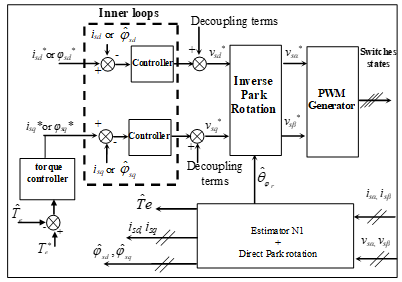Figure 1. Voltage inverter based DRFOC drive

The estimator N1 estimates the stator flux components in the stationary reference frame (α, β)s :

${{\hat{\phi }}_{s\alpha }}=\text{ }\int{({{v}_{s\alpha }}-}{{R}_{s}}\text{ }{{i}_{s\alpha }}\text{)}dt,\text{ }{{\hat{\phi }}_{s\beta }}=\text{ }\int{({{v}_{s\beta }}-}{{R}_{s}}\text{ }{{i}_{s\beta }})dt$ (6)

Then, the rotor flux components are estimated as given below:

${{\hat{\phi }}_{r\alpha }}=\frac{{{L}_{r}}}{{{L}_{m}}}\text{ (}{{\hat{\phi }}_{s\alpha }}-\sigma {{L}_{s}}\text{ }{{i}_{s\alpha }}\text{), }{{\hat{\phi }}_{r\beta }}=\frac{{{L}_{r}}}{{{L}_{m}}}\text{ (}{{\hat{\phi }}_{s\beta }}-\sigma {{L}_{s}}\text{ }{{i}_{s\beta }}\text{)}$  (7)

The rotor flux position with respect to the stator reference frame and the electromagnetic torque are estimated respectively by:

${{\hat{\theta }}_{{{\phi }_{r}}}}=arctg(\frac{{{{\hat{\phi }}}_{r\beta }}}{{{{\hat{\phi }}}_{r\alpha }}})$ (8)

${{\hat{T}}_{e}}=p\frac{{{L}_{m}}}{{{L}_{r}}}({{\hat{\phi }}_{r\alpha }}{{i}_{s\beta }}-{{\hat{\phi }}_{r\beta }}{{i}_{s\alpha }})=p\frac{{{L}_{m}}}{\sigma {{L}_{r}}{{L}_{s}}}({{\hat{\phi }}_{r\alpha }}{{\hat{\phi }}_{s\beta }}-{{\hat{\phi }}_{r\beta }}{{\hat{\phi }}_{s\alpha }})$ (9)

3. Voltage Inverter Based DRFOC Diagram Simplification

Actually, voltage inverter based DRFOC scheme can be significantly simplified if stator flux control is achieved in the stator reference frame (α, β)s. In fact, in this frame the stator resistance is often neglected. Also, the direct Park rotation could be removed because there is no need to compute direct and quadratic stator flux components. Moreover, in this frame, even the PWM generator block may be removed, as discussed below.

3.1 Controlling stator flux in (α, β)s reference frame and elimination of direct Park rotation

In the (α, β)s reference frame, when the stator resistance is neglected, the expression that links the stator flux and voltage become:

${{v}_{s\alpha }}=\frac{d{{\phi }_{s\alpha }}}{dt}$,${{v}_{s\beta }}=\frac{d{{\phi }_{s\beta }}}{dt}$  (10)

In order to achieve the stator flux control in the (α, β)s reference frame, we use the so-called discrete derivative controllers ,which ensures the following computations :

\left\{ \begin{align} & {{v}_{s{{\alpha }_{k}}}}^{*}=\frac{{{(\Delta {{\phi }_{s\alpha }}_{_{k}})}^{*}}}{{{T}_{s}}}\text{ with:}{{(\Delta {{\phi }_{s\alpha }}_{_{k}})}^{*}}={{\phi }_{s\alpha }}{{_{_{k}}}^{*}}-{{{\hat{\phi }}}_{s\alpha }}_{_{k-1}} \\ & {{v}_{s{{\beta }_{k}}}}^{*}=\frac{{{(\Delta {{\phi }_{s\beta }}_{_{k}})}^{*}}}{{{T}_{s}}}\text{with:}{{(\Delta {{\phi }_{s\beta }}_{_{k}})}^{*}}={{\phi }_{s\beta }}{{_{_{k}}}^{*}}-{{{\hat{\phi }}}_{s\beta }}_{_{k-1}} \\ \end{align} \right.  (11)

where ${{{\hat{\phi }}}_{s\alpha }}_{_{k-1}}$ and ${{{\hat{\phi }}}_{s\beta }}_{_{k-1}}$ represent the estimated stator flux components at the time instant tk-1, and Ts is the used sampling time. Thus, the DRFOC diagram becomes:

## figure_2.pngFigure 2. DRFOC drive diagram in (α, β)s

We note that this technique is very close to the DTC-SVM. The only difference is the angle used for reverse Park rotation.  The DTC-SVM uses the angle of the stator flux vector whereas here it is rather the rotor flux one.

3.2 Elimination of the PWM generator

Actually, in the stationary reference frame (α, β)s, it is possible to achieve stator flux control through hysteresis controllers as detailed in . The diagram of the DRFOC could be illustrated as given by figure 3, where, Table I presents the switching table and the two chosen controllers are shown respectively in figures 4 and 5.

In fact, according to the effect brought by each voltage vector to the stator flux components in the (α, β)s reference frame, a switching table I is summarized as follow :

## figure_3.png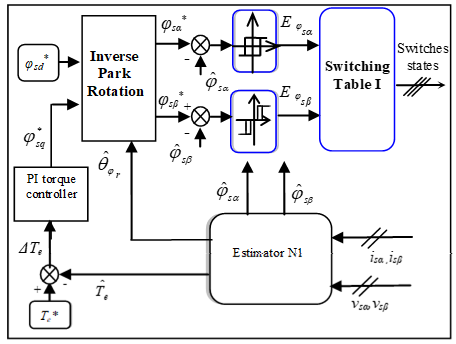Figure 3. DRFOC drive diagram in (α, β)s using a switching table

## figure_4.png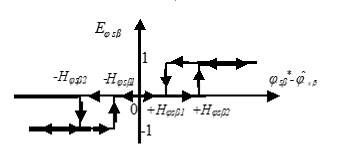Figure 4. DRFOC drive diagram in (α, β)s using a switching table

## figure_5.pngFigure 5. φsα hysteresis controller

Table 1. Switching table I

 Eφsα 1 -1 Eφsβ 1 0 -1 1 0 -1 $\bar{v}_s$ $\bar{v}_{s_2}$ $\bar{v}_{s_1}$ $\bar{v}_{s_6}$ $\bar{v}_{s_3}$ $\bar{v}_{s_4}$ $\bar{v}_{s_5}$

Eφ  =1 (Eφ=1) means that φsαsβ) should increase, Eφ  =-1 (Eφ=-1 ) means that φsαsβ)  should decrease, and Eφ =0 means that φsβ should be kept unchanged. While Hφ and Hφ represent respectively the half of the desired φsα and φsβ hysteresis bounds.

From figure 3, it’s clear that the DRFOC diagram, could be achieved by controlling the stator flux components expressed in the (α, β)s reference frame using two hysteresis controllers and a simple switching table (only six rules).

4. Experimental Results

In order to validate the proposed DRFOC (figure 3), in terms of torque dynamics and both flux and stator current distortions, practical tests with various torque references were carried out. The realized experimental test rig is shown in figure 6. Indeed, it consists of:

1. An IGBTs static voltage inverter (AC/DC/AC) that includes two current sensors (0.1 V for 1 A),
2. Two voltage sensors (1 V for 120 V),
3. A 1.5 kW IM mechanically linked to a 1.5 kW DC generator. The parameters of the used IM are presented in Table II.
4. A DSPace 1104 board based on a 250 MHz 603-PowerPC-64-bit processor is used for data acquisition, IM states estimation and algorithm computing,
5. A control PC where the DSPace is plugged,
6. A/D converters (12-bit, 800 ns),
7. An interface that converts the IGBTs control signals from TTL to CMOS,
8. A/D converters and
9. A 2 kW resistive bank (box switches) which is supplied by the DC generator and was adjusted in such a way that the produced load torque reaches the nominal IM torque at nominal speed.

After building the proposed DRFOC real-time Simulink-blocks, the C code is automatically generated by the Real-Time Workshop in conjunction with the DSPace’s Real-Time Interface. The Real-Time Application is downloaded and executed in the DSPace’s global memory (32 MB, SDRAM). To monitor and save all the experiment data, we use the ControlDesk which is a DSPace’s experiment software.

## figure_6.png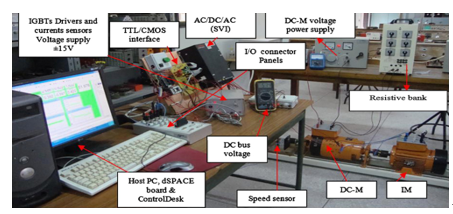Figure 6. The experimental platform

Table 2. The IM parameters values

 p 2 P 1.5 kW Rs 4.75 Ω Isn 4.2 A Rr 1.2 Ω Vsn 380 V Ls 0.4 H Ten 10 N.m Lr 0.072 H ωn 175 rad/s Lm 0.163 H f=fIM+fload 25 ×10-4 Kg.m2/sec J=JIM +Jload 0.025 Kg.m2 Ten 10 N.m

In order to limit torque and stator flux ripples within ±2.5% of their nominal values, the synthesis of DRFOC controller has been done as detailed in . The adopted controllers’ parameters are given as follow:

$Hφ_{sα}=Hφsβ1=1.38×10^{-4}Wb$ (12)

$Hφ_{sβ2}=1.5×10^{-2}Wb$ (13)

${{k}_{p}}=\text{0}\text{.12}$ (14)

${{k}_{i}}=4.44$ (15)

The torque reference sequence lasts 35 (sec): Te * = [8, 5, 2, -6, -2, 6] N.m (figure 7). It is clear that this sequence covers different operating torque points (large steps, positive, negative, small and medium torque values). After a first implementation, we have obtained a computation time Tc = 50 (μsec). So we have chosen to execute the control with a sampling time Ts = 60 (μsec). The obtained experimental results are presented in the following figures:

## figure_7.png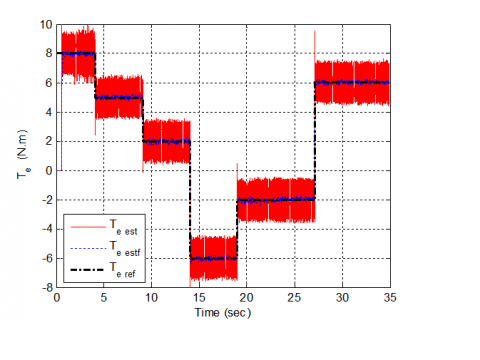Figure 7. Estimated (Te est), reference (Te ref) and filtered (Te estf) torques

## figure_8.pngFigure 8. Stator flux vector locus

## figure_9.pngFigure 9. Stator flux: d-axis component (Fluxsd ref), q-axis component (Fluxs qref), reference magnitude (Fluxs ref) and estimated magnitude (Fluxs est)

## figure_10.pngFigure 10. Stator flux α-axis component: (a) reference and estimated, (b) zoomed figure

## figure_11.png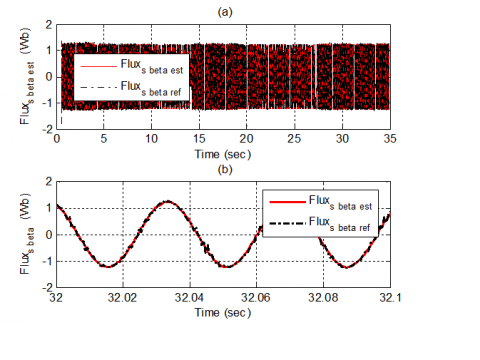Figure 11. Stator flux β-axis component: (a) Reference and estimated, (b) zoomed figure

## figure_12.pngFigure 12. Stator current (a) measured, (b) zoomed figure

From Figure 7, we clearly see that the filtered estimated torque (dashed line) does not present any error with the use of the proposed DRFOC including a PI torque controller. Because the used sampling time is not small enough, we note that the torque ripple goes beyond the set hysteresis bounds (±0.25 N.m). Further, we note that the use of hysteresis stator flux controllers has improved the DRFOC torque dynamic that has become comparable to that of the classical DTC. The figure 8 shows that the flux vector locus presents few distortions, few ripples and closer circle shape.

From Figure 9, it’s clear that the estimated stator flux magnitude follows the desired magnitude reference and presents few ripples. For the same raison as for the torque, the magnitude flux ripples go beyond the set limits (±0.03 Wb). Less distortion could be achieved if less sampling time could have been used. The same figure shows also that the stator flux reference q-component follows exactly the torque shape, while, the d-component remains constant.

Figures 10 and 11 present the stator flux components waveforms in the stator reference frame. Indeed, Figures 10(b) and 11(b) show that the estimated values of these two components follows exactly their references and present almost-perfect sinusoidal forms. Thereby, even the stator current shape presents a sinusoidal form, as shown in Figure 12.

5. Conclusions

This paper has presented a novel approach to achieve DRFOC. In fact, from the possibility of realizing the DRFOC through stator flux inner loops performed in the stationary reference frame, we have confirmed theoretically and practically that this control can be achieved using: i) a linear torque controller, ii) Park inverse rotation, iii) a reduced switching table, iv) two hysteresis controller and v) a rotor flux angle and stator flux components estimator. In this case, DRFOC achieves better torque dynamic. Our practical realization was performed on a 1.5 kW IM torque drives.

The proposed control design has improved the torque dynamics and proved that the DRFOC could be achieved without using any current inner loop and no PWM bloc.

In this paper we have shown that the DRFOC could achieve the DTC dynamics. As a perspective, comparison with classical DRFOC and classical DTC, in terms of dynamic and steady state performance, might be investigated. Further, a comparison of THD/Torque ripple performance should be realized.

Nomenclature
 vs, is Stator voltage and current. φs, φr Stator and rotor flux. θφs ,θφr Positions of stator and rotor flux in the stator frame. ω Mechanical rotor speed. 2.p number of poles. Te Electromagnetic torque. Tr Rotor time constant. Rs Stator resistance. Ls ,Lr ,Lm Stator, rotor and mutual inductances. σ Total leakage factor. H(.) Denotes hysteresis bound for a chosen variable. E(.) Denotes logical decision for a chosen variable. (.)* Denotes a reference value. (^) Denotes an estimated value. (.)α,β Denotes α and β axis-components for a chosen variable. (.)d,q Denotes d and q axis-components for a chosen variable.

References

 Roubache T, Chaouch S, Natï-Sadï MS. (2018). Comparative study between luenberger observer and extended kalman filter for fault-tolerant control of induction motor drive. AMSE IIETA Advances in Modelling and Analysis C 73(2): 29-36. https://doi.org/10.18280/ama_c.730201

 Lokriti A, Salhi I, Doubabi S, Zidani Y. (2013). Induction motor speed drive improvement using fuzzy IP-self-tuning controller A real time implementation. ISA Transactions 52(3): 406-417. https://doi.org/10.1016/j.isatra.2012.11.002

 Belhamdi S, Amar G. (2017) Direct field-oriented control using fuzzy logic type-2 for induction motor with broken rotor bars. AMSE JOURNALS-AMSE IIETA Publication 72(4): 203-212. https://doi.org/10.18280/ama_c.720401

 Lallouani H, Belhamdi S. (2018). Speed control of doubly star induction motor (DSIM) using direct field-oriented control (DFOC) based on fuzzy logic controller (FLC). AMSE IIETA Advances in Modelling and Analysis C 73(4): 128-136. https://doi.org/10.18280/ama_c.730402

 Gastaldini CC, Vieira RP, Azzolin RZ, Grndling HA. (2013). Feedback linearization control with sliding mode speed observer for three-phase induction machines. 2013 Brazilian Power Electronics Conference, pp. 345-349. https://doi.org/10.1109/COBEP.2013.6785138

 Sira-Ramirez H, Gonzalez-Montanez F, Cortes-Romero JA, Luviano-Juarez A. (2013). A robust linear field-oriented voltage control for the induction motor: experimental results. IEEE Transactions on Industrial Electronics 60(8): 3025-3033. https://doi.org/10.1109/TIE.2012.2201430

 Gokdere LU, Simaan MA, Brice CW. (2001). Passivity-based control of saturated induction motors. IEEE Transactions on Industrial Electronics 48(4): 870-872. https://doi.org/10.1109/41.937423

 Amezquita-Brooks L, Liceaga-Castro J, Liceaga-Castro E. (2014). Speed and position controllers using indirect field-oriented control: A classical control approach. IEEE Transactions on Industrial Electronics 60(4): 1928-1943. https://doi.org/10.1109/TIE.2013.2262750

 Belhamdi S, Goléa A. (2015). Direct torque control for induction motor with broken bars using fuzzy logic type-2. AMSE Journals Series: Modelling C 70(1): 15-28.

 Sorchini Z, Krein PT. (2006). Formal derivation of direct torque control for induction machines. IEEE Transactions on Power Electronics 21(5): 1428-1436. https://doi.org/10.1109/TPEL.2006.882086

 Barika SK, Jaladi KK. (2016). Five-phase induction motor DTC-SVM scheme with PI controller and ANN controller. Procedia Technology 25: 816-823. https://doi.org/10.1016/j.protcy.2016.08.184

 Ammar A, Bourek A, Benakcha A. (2017). Nonlinear SVM-DTC for induction motor drive using input-output feedback linearization and high order sliding mode control. ISA Transactions 67: 428-442. https://doi.org/10.1016/j.isatra.2017.01.010

 Costa BLG, Graciola CL, Angélico BA, Goedtel A, Castoldi MF. (2018). Metaheuristics optimization applied to PI controllers tuning of a DTC-SVM drive for three-phase induction motors. Applied Soft Computing 62: 776-788. https://doi.org/10.1016/j.asoc.2017.09.007

 Ammar A, Benakcha A, Bourek A. (2017). Closed loop torque SVM-DTC based on robust super twisting speed controller for induction motor drive with efficiency optimization. International Journal of Hydrogen Energy 42(28): 17940-17952. https://doi.org/10.1016/j.ijhydene.2017.04.034

 Rezgui SE, Mehdi A, Legrioui S, Meddouce H. (2013). IRFOC vs DTC performance comparison analysis. 2013 3rd International Conference on Electric Power and Energy Conversion Systems. https://doi.org/10.1109/EPECS.2013.6713066

 Niu F, Wang K, Li B, Strangas EG. (2016). Comparative evaluation of direct torque control strategies for permanent magnet synchronous machines. IEEE Transactions on Power Electronics 31(2): 2438-2445. https://doi.org/10.1109/TPEL.2015.2421321

 Patil UV, Suryawanshi HM, Renge MM. (2012). Performance comparison of DTC and FOC induction motor drive in five level diode clamped inverter. IEEE-International Conference on Advances in Engineering, Science and Management (ICAESM -2012), pp. 227-230.

 Korkmaz F, Topaloglu I, Cakir MF, Gurbuz R. (2013). Comparative performance evaluation of FOC and DTC controlled PMSM drives. 4th International Conference on Power Engineering, Energy and Electrical Drives, 705-708. https://doi.org/10.1109/PowerEng.2013.6635696

 ElOuanjli N, Derouich A, El-Ghzizal A, Chebabhi A, Taoussi M. (2018). A comparative study between FOC and DTC control of the Doubly Fed Induction Motor (DFIM). 2017 International Conference on Electrical and Information Technologies (ICEIT). https://doi.org/10.1109/EITech.2017.8255302

 Struharňanský Ľ, Vittek J, Makyš P, Ilončiak J. (2017). Vector control techniques for traction drive with induction machines–comparison. Procedia Engineering 192: 851-856. https://doi.org/10.1016/j.proeng.2017.06.147

 Liu CA, Luo Y. (2017). Overview of advanced control strategies for electric machines. Chinese Journal of Electrical Engineering 3(2): 53-61. https://doi.org/10.23919/CJEE.2017.8048412

 Sudheer H, Kodad SF, Sarvesh BC. (2018). Improvements in direct torque control of induction motor for wide range of speed operation using fuzzy logic. Journal of Electrical Systems and Information Technology 5(3): 813-828. https://doi.org/10.1016/j.jesit.2016.12.015

 Fahassa C, Sayouti Y, Akherraz M. (2015). Improvement of the induction motor drive's indirect field oriented control performance by substituting its speed and current controllers with fuzzy logic components. 2015 3rd International Renewable and Sustainable Energy Conference (IRSEC). https://doi.org/10.1016/j.asoc.2017.09.00710.1109/IRSEC.2015.7454928

 Panchade VM, Chile RH, Patre BM. (2013). A survey on sliding mode control strategies for induction motors. Annual Reviews in Control 37(2): 289-307. https://doi.org/10.1016/j.arcontrol.2013.09.008

 Kaimierkowski MP, Dzieniakowski MA, Sulkowski W. (1991). Novel space vector based current controllers for pwm-inverters. IEEE Transaction on Power Electronics 6(1): 158-166. https://doi.org/10.1109/63.65014

 Kazmierkowski MP, Krishnan R, Blaabjerg F. (2002). Control in power electronics: Selected problems.

 Zhang Z, Tang R, Bai B, Xie D. (2010). Novel direct torque control based on space vector modulation with adaptive stator flux observer for induction motors. IEEE Transactions. on Magnetics 46(8): 3133-3136. https://doi.org/10.1109/TMAG.2010.2051142

 Lokriti A, Salhi I, Doubabi S. (2015). IM direct torque control with no ﬂux distortion and no static torque error. ISA Transactions 59: 256-267. https://doi.org/10.1016/j.isatra.2015.08.014